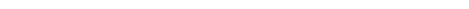### 15.4 Working with Java arrays

Java arrays are represented inside Lisp by a jobject or an instance of standard-java-object, like any other Java object. The function java-array-element-type returns the element type of a Java array or `nil` if it is not an array, and it is fast enough that it can be used as a predicate to determine whether a jobject represents an array.

java-array-length returns the length of a Java array.

java-primitive-array-element-type and java-object-array-element-type return the same values as java-array-element-type for an array of primitive type or an array of non-primitive type respectively, otherwise they return `nil`. They are fast and can be used as predicates to decide whether an array is of primitive type or not.

Java arrays of higher dimensions are represented recursively as vectors of vectors, which affects the way you use the accessors.

#### 15.4.1 Accessing a single element

The accessor jvref can be used to get and set (with `cl:setf`) the value in a Java "Vector" (that is, a one-dimensional array). For a multi-dimensional array, jvref gets and sets the first level "Vector", in other words it returns another array of one less dimension.

jaref can be used to get and set elements of arrays with any number of dimensions. If the number of dimensions given is less than the rank of the array, it gets or sets the corresponding sub-array.

Both jvref and jaref can be used to access arrays of any type. jvref is slightly faster, and does not allow passing wrong number of arguments.

Note: when accessing an element of a multi-dimensional array, jaref needs to get the sub-arrays for the sub-dimensions. This means it is relatively inefficient when used to access elements in the same sub-array. It is more efficient to get the sub-array and access it. For example, instead of

`(dotimes (z 10)`
`  (do-something (jaref java-array 3 4 z)))`

use

`(let ((sub-array (jaref java-array 3 4)))`
`  (dotimes (z 10)`
`    (do-something (jvref sub-array z))))`

Assuming `java-array` is not a primitive array, it is even better to use the multiple access functions:

`(let ((sub-array (jaref java-array 3 4)))`
`  (map-java-object-array 'do-something sub-array :end 10))`

#### 15.4.2 Making Java arrays

The function make-java-array is used to make Java arrays of any rank and type. It takes as first argument a class specifier, followed by the dimension(s). The class specifier specifies the type of the elements in the array, which may be any type (both primitives and proper classes).

It is also possible to create primitive arrays with data copied from Lisp arrays using lisp-array-to-primitive-array.

#### 15.4.3 Multiple access functions

The multiple access functions are used to access elements in one-dimensional arrays ("Vectors"). They are much more efficient than accessing each element separately.

Multiple access of primitive and non-primitives is done in a different way: non-primitive arrays are accessed by map-java-object-array, which maps a function on the objects in the array. Primitive arrays are accessed by primitive-array-to-lisp-array and lisp-array-to-primitive-array (copy to or from a Lisp array) or get-primitive-array-region and set-primitive-array-region (copy to or from a foreign array). `String` arrays are regarded as `Object` arrays for this distinction.

map-java-object-array maps a function across an array. It has keyword arguments to control the actual operation, including specifying the range and direction, writing back the result of the call, and collecting the values. When called on multi-dimensional arrays, map-java-object-array accesses the top level elements, that is sub-arrays of one less dimension.

primitive-array-to-lisp-array and lisp-array-to-primitive-array take a Java primitive array or a Lisp array respectively, and copy the elements to a Lisp array or Java primitive array. Both functions can copy into an existing array or create the array themselves. Keyword arguments allow you to specify the range to copy.

Both primitive-array-to-lisp-array and lisp-array-to-primitive-array require the Lisp array element type to match exactly the Java array element type. The corresponding types are:

######Java array element type

Lisp array element type

`:int`

`(signed-byte 32)`

`:long `

`(signed-byte 64)`

`:short`

`(signed-byte 16)`

`:byte`

`(signed-byte 8)`

`:double`

`double-float`

`:single`

`single-float`

`:char`

`(unsigned-byte 16)`

`:boolean`

`(unsigned-byte 8)`

get-primitive-array-region and set-primitive-array-region take a primitive array and copy part of it to or from a foreign array ("buffer"), which is passed as an FLI pointer.

LispWorks User Guide and Reference Manual - 13 Feb 2015